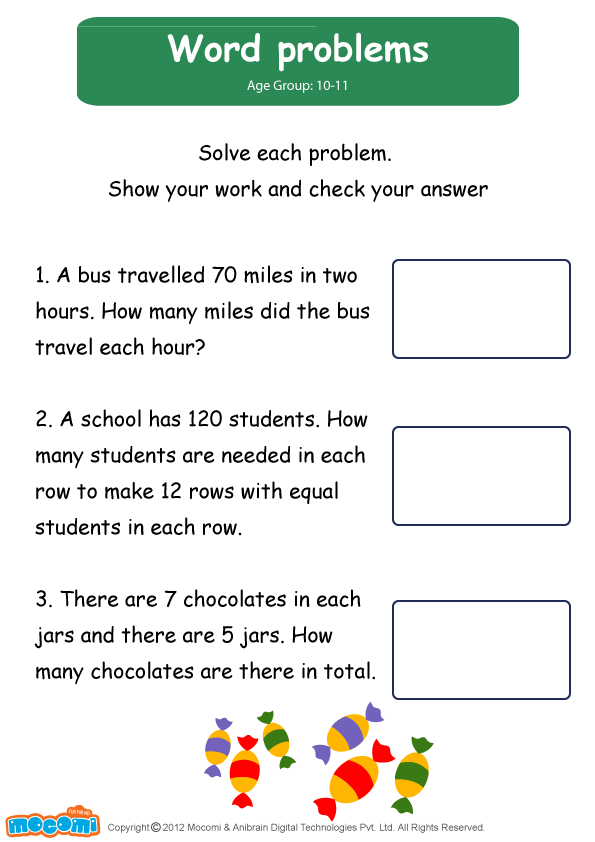• 0
• 0
• 0
• 0
• Embed CodePrevious Article
Next Article

# Word Problems Worksheet

Maths | 5-9 yrs | Learning Pod

Word Problems Worksheet – Sharpen your skills in math word problems with this math worksheet for kids which can also be downloaded for free.

## Word Problems Worksheet:

Solve each problem. Show your work and check your answer:

1. A bus travelled 70 miles in two hours. How many miles did the bus travel each hour? __________
2. A school has 120 students. How many students are needed in each row to make 12 rows with equal students in each row? __________
3. There are 7 chocolates in each jars and there are 5 jars. How many chocolates are there in total? __________

For more interesting Maths worksheets and lessons, go to : https://mocomi.com/learn/maths/

## RECOMMENDED ARTICLES

1.harsha
2.Maha